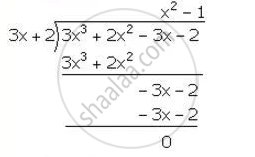Share

(3x + 2) is a Factor of 3x3 + 2x2 – 3x – 2. Hence, Factorise the Expression 3x3 + 2x2 – 3x – 2 Completely. - ICSE Class 10 - Mathematics

Question

(3x + 2) is a factor of 3x3 + 2x2 – 3x – 2. Hence, factorise the expression 3x3 + 2x2 – 3x – 2 completely.

Solution

Let f(x)=3x^3+2x^2-3x-2

3x+2=0  ⇒ x=(-2)/3

∴ Remainder = f ((-2)/3)

=3((-2)/3)^3+2((-2)/3)^2-3((-2)/3)-2

= (-8)/9+8/9+2-2

= 0

Hence,(3x+2) is a factor of f (x)

Now, we have:∴ 3x^3+2x^2-3x-2=(3x+2)(x^2-1)=(3x+2)(x+1)(x-1)

Is there an error in this question or solution?

Video TutorialsVIEW ALL 

Solution (3x + 2) is a Factor of 3x3 + 2x2 – 3x – 2. Hence, Factorise the Expression 3x3 + 2x2 – 3x – 2 Completely. Concept: Factor Theorem.
S